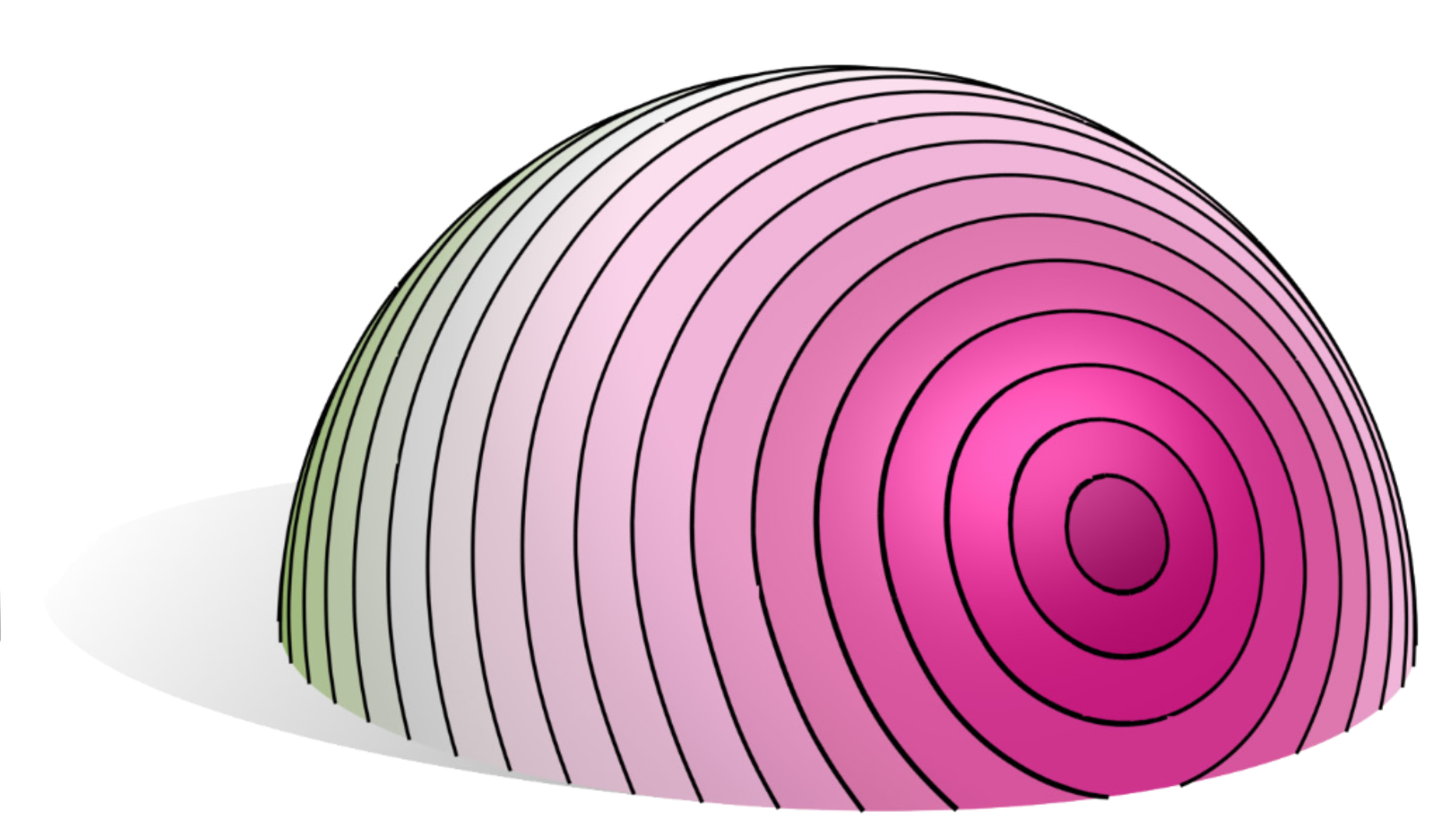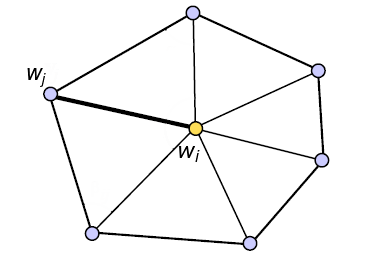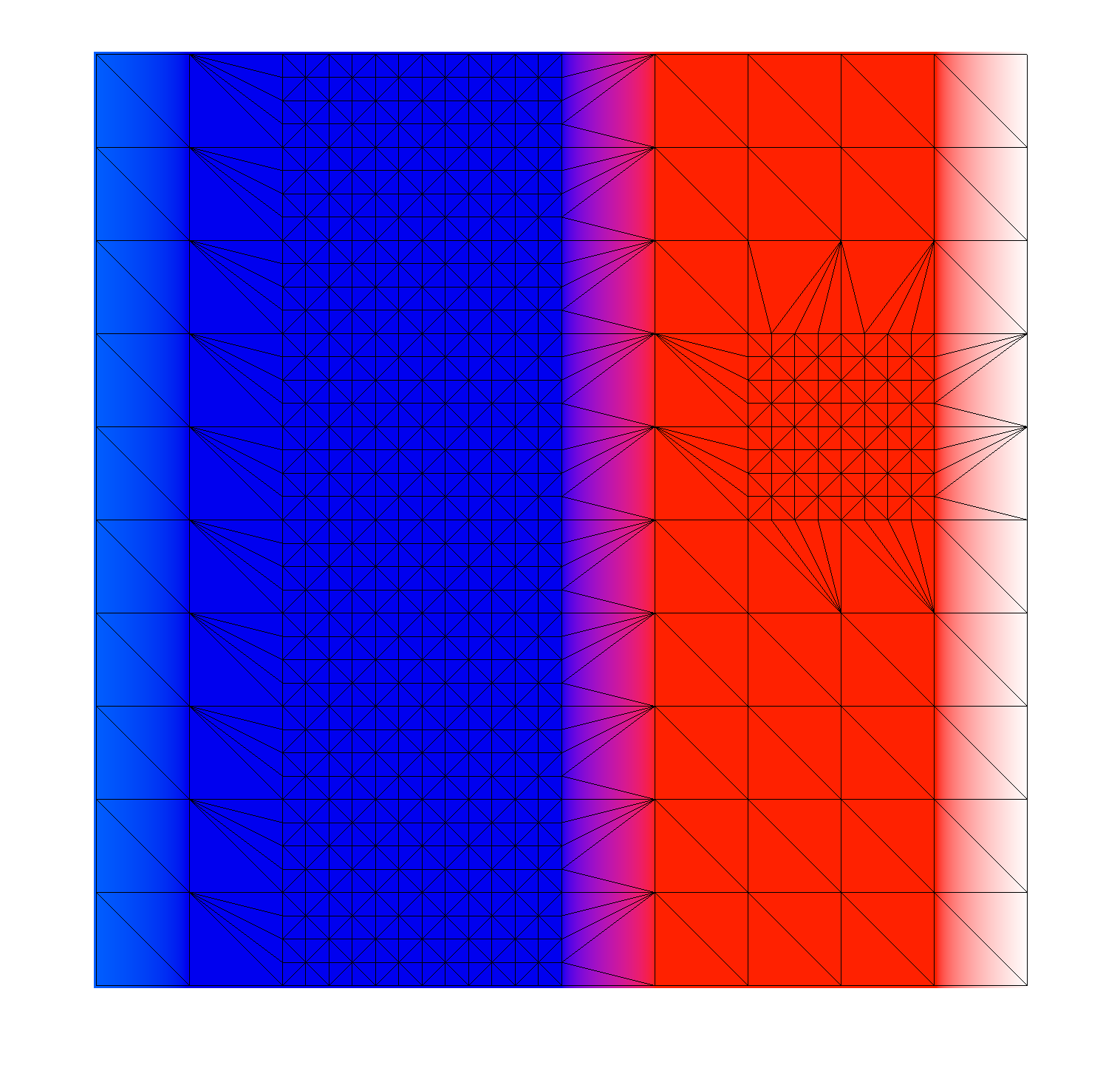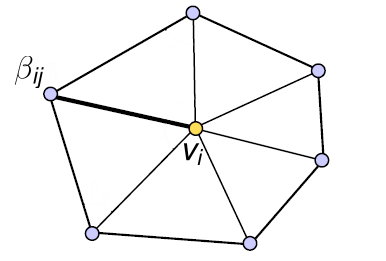# Diffusing / smoothing weight map over a triangular mesh.Showcasing simple procedures with C++ code to smooth / diffuse per vertex weights over a triangle mesh.

Say you associate each vertex of a mesh $$v_i$$ with a real value $$w_i$$, it is possible to diffuse or smooth this per vertex weight map over the surface of the mesh.## Uniform diffusion

Let's consider the direct neighbors of the weight $$w_i$$, namely the weights $$\{\cdots, w_{j}, \cdots \}$$:For every vertices we can average the values $$\{\cdots, w_{j}, \cdots \}$$ surrounding vertex $$i$$:

$$w_i' = \sum\limits_{{j \in \mathcal N(i)}} { \frac{w_{j}}{|\mathcal N(i)|} }$$

Where $$\mathcal N(i)$$ is the set of vertices adjacent to $$i$$ and $$| \mathcal N(i)|$$ the number of vertices connected to $$i$$ (i.e. its valence). Notice here we ignore altogether the old value $$w_i$$ and directly replace it with its new value $$w_i'$$. You need two buffers $$\mathbf w = \{w_1, \cdots, w_n \}$$ and $$\mathbf w' = \{w_1', \cdots, w_n' \}$$. Once all the new values $$\mathbf w'$$ are computed you can swap the buffers and repeat the process to further smooth out the results.Here we set constant values on the left (=0) and right side (=1) of the plane and diffuse the interior values. After diffusion the values will smoothly progress in the interval $$[0, 1]$$.

If you wish to slow down the diffusion process this is easily performed by linearly interpolating the old value $$w_i$$ with the new one:

$$w_i' = w_i . (1-t) + \left ( \sum\limits_{{j \in \mathcal N(i)}} { \frac{w_{j}}{|\mathcal N(i)|} } \right ) . t$$

Where $$t \in [0, 1]$$ is your interpolation factor.

While values are effectively smoothed out you can notice the result is not symmetrical. This is because we completely ignore the fact triangles have different size and shape. Intuitively a neighbor $$v_{j}$$ far from $$v_i$$ should contribute less than a closer neighbor when we average the values.

## Weighted diffusion

To take into account the mesh irregularities we need to introduce barycentric weights $$\mathbf \beta$$. For every vertices $$v_i$$ we associate a set of weights $$\{ \cdots, \beta_{ij}, \cdots \}$$ corresponding to each direct neighbor:$$\mathbf{ \beta }= \left \{ \{ \beta_{1 1}, \cdots, \beta_{1 |\mathcal N(1)| } \}, \cdots,\{ \cdots, \beta_{ij},\cdots\} , \cdots, \{ \beta_{i 1}, \cdots, \beta_{i |\mathcal N(i)| } \} \right \}$$

We now do a weighted average of the neighboring values:
$$w_i' = w_i (1-t) + \left ( \frac{ \sum\limits_{{j \in \mathcal N(i)}} w_{ij} \beta_{ij} }{ \sum\limits_{{j \in \mathcal N(i)}} { \beta_{ij} } } \right ) . t$$

Notice that if we set $$\beta_{ij} = 1$$ the formula simplifies to the uniform case. Carefully setting up the weights $$\beta_{ij}$$ will allow us to take into account irregular triangle distribution and we can obtain perfectly symmetrical results:This result was obtain with the so called cotangent weights quite popular in geometry processing. If you iterate the process enough times the values will be all equal on the vertical axis $$y$$. On the horizontal axis $$x$$ from the right to the left side values will increase linearly i.e. $$f(x) = x . (1 / hlength)$$.

As a simpler alternative you could use edge length but it will produce more irregular results:

$$\beta_{ij} = \frac{\sum\limits_{k \in \mathcal N(i)} {\|v_i - v_k\| } }{{\|v_i - v_j\| }}$$

## Code

You will also need to take a look at my blog post on cotangent weights to fully take advantage of the code below.

/// @param weights : per vertex weight map to smooth.
void smooth(const std::vector& weight_map,
const Mesh& mesh)
{
enum Smoothing_type {
eUNIFORM = 0,
eEDGE_LENGTH,
eCOTAN
};

Smoothing_type type = eCOTAN;
float t = 1.0f;

// For every vertex compute a weight for it's direct neighbors:
// per_edge_weights[vertex_i][neighbor_of_i] = w_ij
std::vector< std::vector > per_edge_weights( mesh.size() );

switch(type){
case(eEDGE_LENGTH):
{
for(unsigned vertex_i : mesh)
{
Vec3 pos_i = mesh.vertex(vertex_i);

unsigned nb_neigh = mesh.nb_neighbors(vertex_i);
per_edge_weights[vertex_i].resize(nb_neigh);

float total_length = 0.0f;
for(unsigned edge_j = 0; edge_j < nb_neigh; edge_j++)
{
int vertex_j = mesh.first_ring()[vertex_i][edge_j];
Vec3 pos_j = mesh.vertex(vertex_j);

float len = (pos_i - pos_j).norm();
total_length += len;
per_edge_weights[vertex_i][edge_j] = len;
}

for(unsigned edge_j = 0; edge_j < nb_neigh; edge_j++)
{
per_edge_weights[vertex_i][edge_j] = total_length / per_edge_weights[vertex_i][edge_j];
}
}
}break;
case(eCOTAN):
{
t = 0.9f; //< otherwise the convergence is not stable
for(unsigned vertex_i : mesh)
{
unsigned nb_neigh = mesh.nb_neighbors(vertex_i);
per_edge_weights[vertex_i].resize(nb_neigh);
float total_weights = 0.0f;
for(unsigned edge_j = 0; edge_j < nb_neigh; edge_j++)
{
// For this function please see my article on cotangent weights
float wij = laplacian_cotan_weight(mesh, vertex_i, edge_j);

per_edge_weights[vertex_i][edge_j] = wij;
total_weights += wij;
}
}
}break;
case(eUNIFORM):
default:
{
t = 1.0f; // With this one you can safely smooth full throttle :)
for(unsigned vertex_i : mesh)
{
unsigned nb_neigh = mesh.nb_neighbors(vertex_i);
per_edge_weights[vertex_i].resize(nb_neigh);
for(unsigned edge_j = 0; edge_j < nb_neigh; edge_j++)
per_edge_weights[vertex_i][edge_j] = 1.0f;
}
}break;
}

// ---------------------------------
// Diffuse weight map:
// ---------------------------------

std::vector new_weight_map( mesh.size() );

for(int iter = 0; iter < nb_iter; iter++)
{
// For all vertices
for(unsigned vertex_i : mesh)
{
float weight_sum = 0.0f;

// For all vertices' neighborhoods sum the weight of each bone
unsigned nb_neigh = mesh.nb_neighbors(vertex_i);
float sum_wij = 0.0f;
for(unsigned edge_j = 0; edge_j < nb_neigh; edge_j++)
{
int vertex_j = mesh.first_ring()[vertex_i][edge_j];

float wij = per_edge_weights[vertex_i][edge_j];
sum_wij += wij;
weight_sum += weight_map[vertex_j] * wij;
}

float central_weight = weight_map[vertex_i];

// is_locked_value() could for instance return true for:
// (v < 0.0f and v > 1.0f) to only smooth values in [ 0.0f, 1.0f]
if( !is_locked_value(central_weight) ){
float new_weight = (weight_sum / sum_wij);
central_weight = central_weight * (1.0f - t) + new_weight * t;
}

new_weight_map[vertex_i][j] = central_weight;

}// END nb_vert

for(unsigned vertex_i : mesh)
{
weight_map[v_idx].swap(new_weight_map[vertex_i]);
new_weight_map[vertex_i].clear();
}

}// END nb_iter
}



Notes:

• you can apply the process to the whole mesh or sub part of the mesh.
• It's possible to use a brush to locally smooth the weight map.
• Notice that when $$t >= 1$$ it will sometimes output erroneous results, in particular for the cotangent weights it is recommended to set something strictly inferior to one to stabilize the convergence.

## Going further

For the curious what we are actually doing here is computing an harmonic function $$f:\mathbb R^2 \rightarrow \mathbb R$$ defined over the surface of a irregular triangle mesh. At each smoothing iteration we are actually computing one Jacobi iteration to solve the linear system of equations $$\Delta f = 0$$. If you set values to form a closed region and iterate enough times you will effectively solve the system completely and compute an harmonic weight map (it is however the slowest way to do so).

If this does not make any sens to you but feel the irrepressible need to know, take a look at my tutorials on harmonic weights. You will see that you can easily smooth weights on regular 2D grids (i.e. a texture) or 3D grids (voxels).

### Data smoothing